+

# Determining Intercepts

##### Rating:
(3)
• (1)
• (0)
• (0)
• (0)
• (2)
Author: Sophia Tutorial
##### Description:

Determine the x-intercept and y-intercept of an linear equation.

(more)
Tutorial
what's covered
1. Determining Intercepts on a Graph
2. Determining Intercepts from a Table
3. Determining Intercepts from an Equation

# 1. Determining Intercepts on a Graph

When we talk about a line's intercepts, we most often mean the x-intercept and the y-intercept. These are locations where the line intercepts or crosses the x- or y- axis. A more formal definition is as follows:

terms to know
x-intercept
where a line or curve intersects the x-axis, at y = 0
y-intercept
where a line or curve intersects the y-axis, at x = 0

Below is the graph of a line, with both intercepts shown: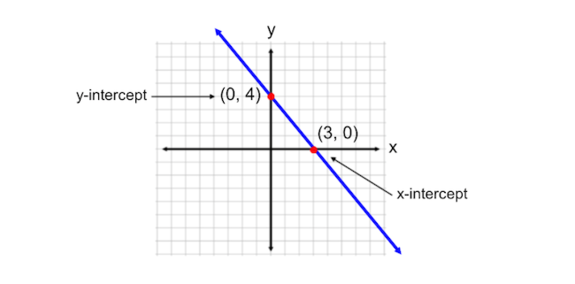Notice that the x-intercept always has a y-coordinate of 0, and the y-intercept always has an x-coordinate of 0.

# 2. Determining Intercepts from a Table

Keeping the above note in mind, we can easily identify intercepts from a table of x and y values when we notice that one of the coordinates is zero! Take a look:

x y
-2 18
0 12
2 6
4 0
6 -6

We see that the second pair (0, 12) contains a zero. In this coordinate pair, the x-coordinate is zero, which means this represents a y-intercept. The y-intercept of the line is at the point (0, 12). We also notice a zero in the coordinate pair (4, 0). In this case, the y-coordinate is zero, so this is the x-intercept. The line crosses the x-axis at the point (4, 0).

# 3. Determining Intercepts from an Equation

How can we use the equation of a line to find its x– and y– intercepts? A common form of a line is in slope-intercept form: y = mx+b. This form is called slope-intercept form, because b represents the y-coordinate of the y-intercept (and we know that its x-coordinate is 0). We can also use the equation in this form to find the x-intercept. Since x-intercepts have a y-coordinate of 0, we set the equation equal to zero and solve for x. This is shown in the example below: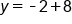Our Equation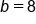When we have the equation y=mx+b, the variable b is the y-coordinate of the y-intercept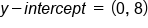For the y-intercept, the x-coordinate is always 0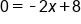To find the x-intercept, plug 0 in for y as the y-coordinate is always 0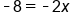Subtract -8 from both sides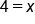Divide by -2. This is the x-coordinate of the x-intercept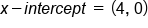For the x-intercept, the y-coordinate is always 0

For the equation y = -2x + 8, the y-intercept is at (0,8) and the x-intercept is at (4,0).

summary
When determining intercepts on a graph, the x-intercept is where a line or a curve intersects the x-axis. It is also where y equals 0 and is written as (x, 0) as a coordinate pair. The y-intercept is where a line or curve intersects the y-axis. It is also where x equals 0 and is written as (0, y) as a coordinate pair. When determining intercepts from a table, we look for instances where x equals 0 or y equals 0. When determining intercepts from an equation, the variable b represents the y-coordinate of the y-intercept. To find the x-intercept, plug in 0 for y and solve for x.
Terms to Know
x-intercept

the location on a graph where a line or curve intersects the x-axis: (x, 0)

y-intercept

the location on a graph where a line or curve intersects the y-axis: (0, y)

Rating Header